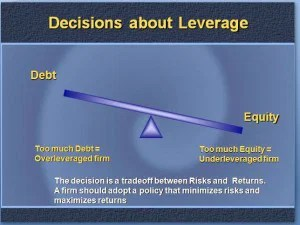# Solvency Ratio or Leverage Ratio

Standard

SOLVENCY OR LEVERAGE RATIOS

Long-term solvency ratios analyze the long-term financial position of the organization. Bankers and creditors are interested in the liquidity of the firm, whereas shareholders, debenture holders and financial institutions are concerned with the long term prosperity of the firm. There are thus two aspects of the long-term solvency of a firm.

1. Ability to repay the principal amount when due
2. Regular payment of the interest.The ratio is based on the relationship between borrowed funds and owner’s capital it is computed from the balance sheet, the second type is calculated from the profit and loss a/c.  The various solvency ratios are

1.  Debt equity ratio
2. Debt to total capital ratio
3. Proprietary (Equity) ratio
4. Fixed assets to net worth ratio
5. Fixed assets to long term funds ratio
6. Debt service (Interest coverage) ratio

1.  DEBT EQUITY RATIO OR EXTERNAL – INTERNAL EQUITY RATIO

Debt equity ratio shows the relative claims of creditors (Outsiders) and owners (Interest) against the assets of the firm. The relationship between borrowed fund and capital is shown in debt-equity ratio. It can be calculated by dividing outsider funds (Debt) by shareholder funds (Equity)

• Ebt equity ratio = Outsider Funds (Total Debts) /  Shareholder Funds or Equity (or)
• Long-term Debts / Shareholders funds
• Shareholders fund = Preference capital + Equity capital + Reserves & Surplus – Goodwill & Preliminary expenses
• Outsiders funds = Current liabilities + Debentures + Loans

The ideal ratio is 2:1. High ratio means, the claim of creditors is greater than owners and vice-versa

2.  DEBT TO TOTAL CAPITAL RATIO

Debt to total capital ratio =  Total Debts / Total Assets

3.  PROPRIETARY (EQUITY) RATIO

This ratio indicates the proportion of total assets financed by owners.  It is calculated by dividing proprietor (Shareholder) funds by total assets.

Proprietary (equity) ratio = Shareholder funds / Total Tangible assets

The ideal ratio is 1:3. A ratio below 50% may be alarming for creditors, because they incur loss during winding up.

4.  FIXED ASSETS TO NET WORTH RATIO

This ratio establishes the relationship between fixed assets and shareholder funds.  It is calculated by dividing fixed assets by shareholder funds.

Fixed assets to net worth ratio =  Fixed Assets X 100 / Net Worth

The shareholder funds include equity share capital, preference share capital, reserves and surplus including accumulated profits.  However fictitious assets like accumulated deferred expenses etc should be deducted from the total of these items to shareholder funds.  The shareholder funds so calculated are known as net worth of the business.

5.  FIXED ASSETS TO LONG TERM FUNDS RATIO

Fixed assets to long term funds ratio establishes the relationship between fixed assets and long-term funds and is calculated by dividing fixed assets by long term funds.

Fixed assets to long term funds ratio = Fixed Assets X 100 / Long-term Funds

6.  DEBT SERVICE (INTEREST COVERAGE) RATIO

This shows the number of times the earnings of the firms are able to cover the fixed interest liability of the firm.  This ratio therefore is also known as Interest coverage or time interest earned ratio.  It is calculated by dividing the earnings before interest and tax (EBIT) by interest charges on loans.

Debt Service Ratio = Earnings before interest and tax (EBIT) / Interest Charges